Articulo Originalhttps://doi.org/10.22463/0122820X.1856

# Macroscopic energy representation and simulation of a permanent magnet synchronous machine

## Representación Energética Macroscópica y Simulación de una Máquina Síncrona de Imanes Permanentes

#### Juan Diego Correa Casas1* Diego Steven Rayo Villamizar2* Javier Enrique Solano Martínez3*

1*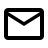Estudiante de Ingeniería Eléctrica, juandiegocorrea.9@gmail.com .Universidad Industrial de Santander, Bucaramanga, Colombia.

2*Estudiante de Ingeniería Eléctrica, diegostevenrayo@gmail.com .Universidad Industrial de Santander, Bucaramanga, Colombia.

3*Ph.D. Electrical engineering, javier.solano@saber.uis.edu.co .ORCID: 0000-0002-6782-5547. Universidad Industrial de Santander, Bucaramanga.

Cómo citar: A. N. Hernández-Durán, W. Gutiérrez-Niño, D. A. Miranda-Mercado, “Electronic localization in Hexagonal CoreShell Nanowires Under External Fields”, Respuestas, vol. 25, no. 3, 154-164, 2020.

© Peer review is the responsibility of the Universidad Francisco de Paula Santander. This is an article under the license CC BY-NC-ND 4.0Approved: July 24, 2020.

Keywords

Control, Permanent Magnet Synchronous Machine, Macroscopic Energetic Representation.

Abstract

This article presents a simulation model of the synchronous permanent magnet machine (MSIP) and its speed control system using the Macroscopic Energetic Representation (REM) formalism. This graphic description allows making a control scheme based on the inversion of the machine model. The models are validated by comparison with the results presented in the literature. To show the utility of the model presented, the simulation results of the machine are presented under different operating conditions and control references.

Palabras claves

Control, Máquina Sincronizada de Imanes Permanentes, Representación Energética Macroscópica.

Resumen

Este artículo presenta un modelo de simulación de la máquina síncrona de imanes permanentes (MSIP) y su sistema de control de velocidad utilizando el formalismo de la Representación Energética Macroscópica (REM). Esta descripción gráfica permite realizar un esquema de control basado en la inversión del modelo de la máquina. Los modelos se validan por comparación con los resultados presentados en la literatura. Para mostrar la utilidad del modelo presentado, se presentan los resultados de simulación de la máquina bajo diferentes condiciones de operación y referencias de control.

Introduction

Permanent magnet synchronous machines have been widely used over time in various industrial applications and electrical processes, such as in research studies on electric vehicles and wind generation , . Its operation is similar to that of a conventional synchronous machine, in which the rotor rotates at the same speed as the rotating magnetic field of the stator, maintaining a phase shift between them equivalent to an angle θ also known as the rotor angle.

There are different ways to study its behavior and in this way evaluate different speed or torque control strategies, such as vector controls, scalar controls, or controls based on non-linear techniques. The Energetic Macroscopic Representation (EMR) , , is a formalism developed for investigations of complex electromechanical conversion systems.

This functional description is a graphical and multi-physical formalism that allows highlighting the properties of each subsystem and allows the understanding of each one of them separately, replacing any functional block (See appendix) and coupling it to the general system without having to modify the whole of the set , based on the principle of action and reaction, and the principle of causality. The EMR control methodology is based on specific investment rules.

Materials and methods

The dynamic model of the MSIP used is the one proposed in , . For this, it is assumed that the effects produced by the stator grooves can be neglected, as well as the losses in the iron and the saturation of the magnetic material. For simplicity, the model will be obtained for a permanent magnet machine, assuming that the air gap has uniform reluctance. If it is considered that the windings of the three phases are symmetrical (the three phases have the same value of inductance and resistance), and also the currents are balanced (ia + ib + ic = 0), the electrical equations of the stator can be written as,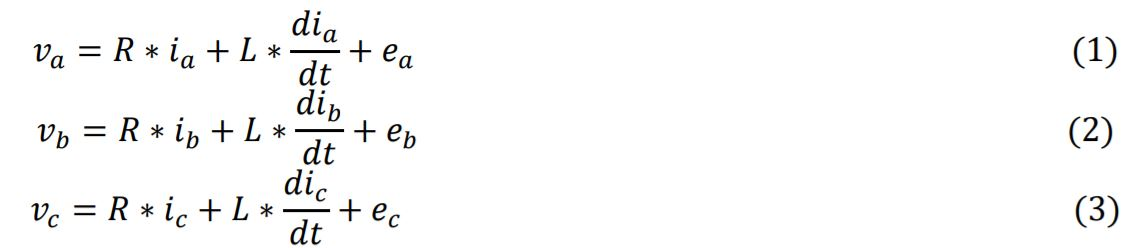Where:

va, vb, and vc are the stator voltages (V).

ia, ib, and ic are the stator currents (A).

ea, eb, and ec are the emf induced in each phase of the stator (V).

The constants R and L are the resistance (Ω) and inductance (H) of the stator, respectively.

The dynamic model of an MSIP can be represented by a new set of variables using the Park transformation [P (θ)], which refers the variables to a stationary frame of reference dq [1-2], that is,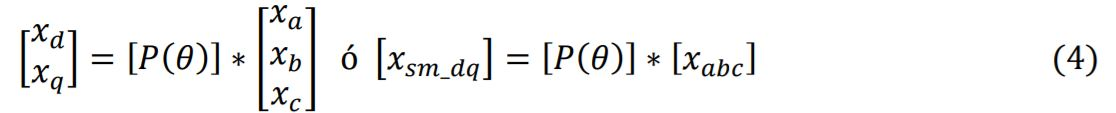Being x arbitrary variables y,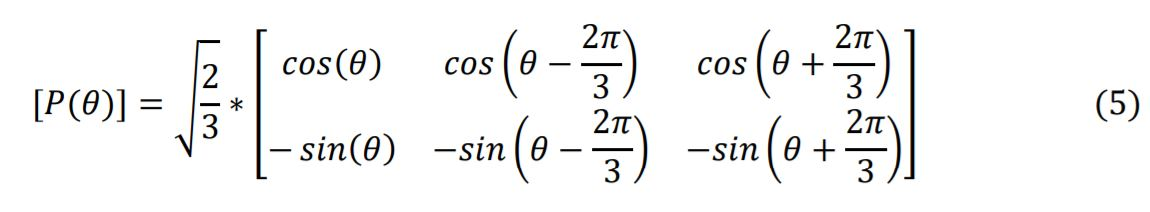Where:

𝜃 is the phase between the stator and the rotor, which can be obtained from the shaft speed: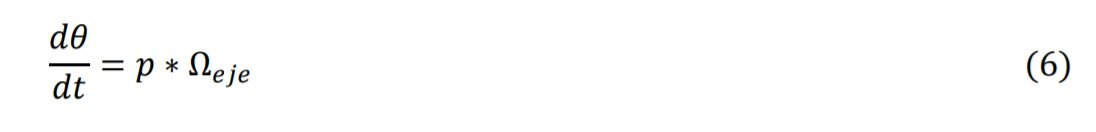Where:

p is the number of pole pairs.

eje is the speed of rotation on the shaft.

In the stationary reference frame dq, the equivalent machine is mainly composed of an accumulation element and an electromechanical converter as shown in Figure 1. Equivalent inductances (armature windings) produce the machine currents 𝑖𝑠𝑚_𝑑𝑞 from stator voltages 𝑣𝑠𝑚_𝑑𝑞 and electromotive forces 𝑒𝑠𝑚_𝑑𝑞 as it is shown in (7).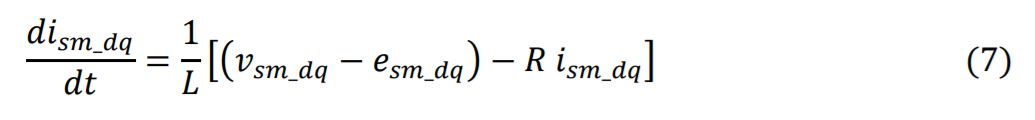In the electromechanical converter, the torque of the machine (8) and the electromotive forces (9) and (10) are produced by the currents of the machine and the speed of rotation of the shaft.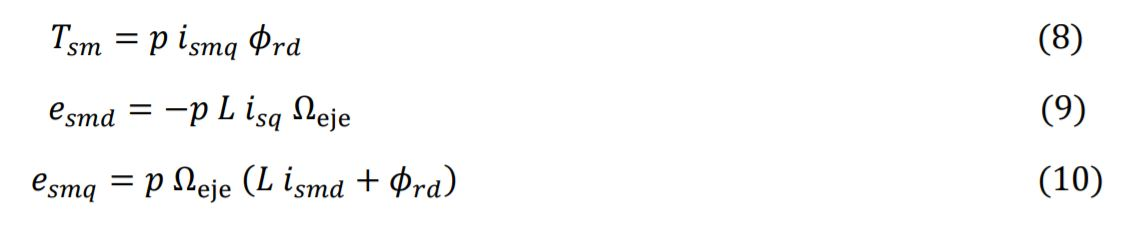Where:

𝑇𝑠𝑚 is the electromagnetic torque of the machine (N.m).

𝜙𝑟𝑑 is the rotor flux induced by the permanent magnets (Wb).

The mechanical equation of the torques of the machine can be seen in the following equation where the speed of the rotor of the machine is determined: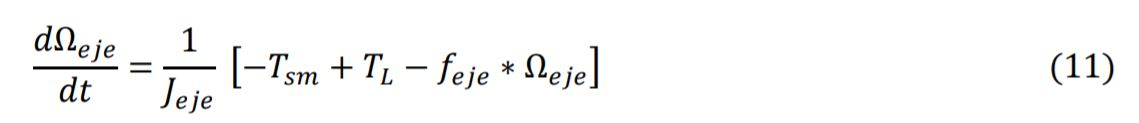Where:

𝐽𝑒𝑗𝑒 and 𝑓𝑒𝑗𝑒 are the moment of inertia (kg.m2) and the coefficient of viscous friction (N.m.s) of the equivalent axis.

EMR basics

Principle of causality:

As in , only integral causality is considered in EMR. This property leads to defining accumulation elements through a time-dependent relationship between its variables, which leads to its output is an integral function of its inputs. Other items are described using non-time dependent relationships. To respect integral causality, specific association rules are defined.

Investment principle:

The control structure of a system is considered as an inversion of the system model: the control has to define the inputs to apply to the system from the desired output (Figure 1). In this method, time-free relationships are reversed directly without control or measurement. Because derived causality is prohibited, a direct reversal of time-dependency relationships is not possible. Therefore, an indirect investment is made using a controller and measurements.

These inversion rules have been extended to EMR (blue pictograms, see appendix): conversion elements are inverted directly, accumulation elements are inverted using a controller (closed-loop control), and coupling devices are inverted using inputs of distribution. It is another way to locate controllers and measurements.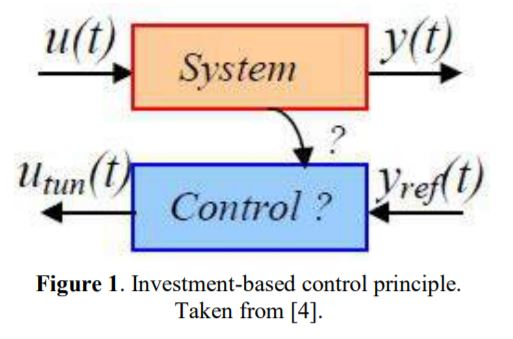As an example of this, the inversion of the voltage-current relationship of the armature winding (7) requires a closed-loop control since it is a time-dependent energy accumulation to obtain the reference voltage 𝑣𝑠𝑚_𝑑𝑞_𝑟𝑒𝑓 from the reference current and its corresponding measurement: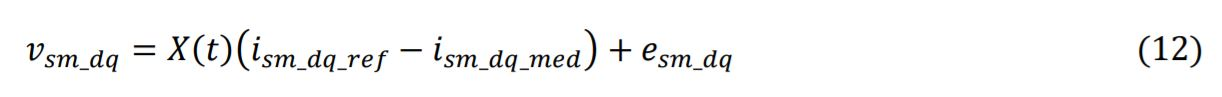Where:

𝑋(𝑡) is the controller which can be P, PI or another type.

A direct inversion of (8) that does not depend on time leads to the reference current 𝑖𝑠𝑚𝑞_𝑟𝑒𝑓 from reference torque 𝑇𝑠𝑚_𝑟𝑒𝑓 as follows: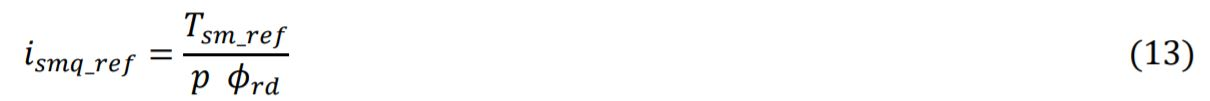Macroscopic Energy Representation of the MSIP

The REM of the MSIP (Figure 2) is made up of the following elements: a direct current source, an inverter, the permanent magnet synchronous machine, and finally, a mechanical load connected to the machine shaft. Each of these elements is represented using the following blocks: a source block (green oval) to represent the electrical source and mechanical load that can be seen as an energy sink, a mono-physical conversion block (orange box) to represent the inverter, an accumulation block (the orange rectangle with line diagonal) to represent the machine windings and finally a multi-physical conversion block to represent the electro-mechanical transformation. The inversion of the machine model is carried out following the rules of the REM.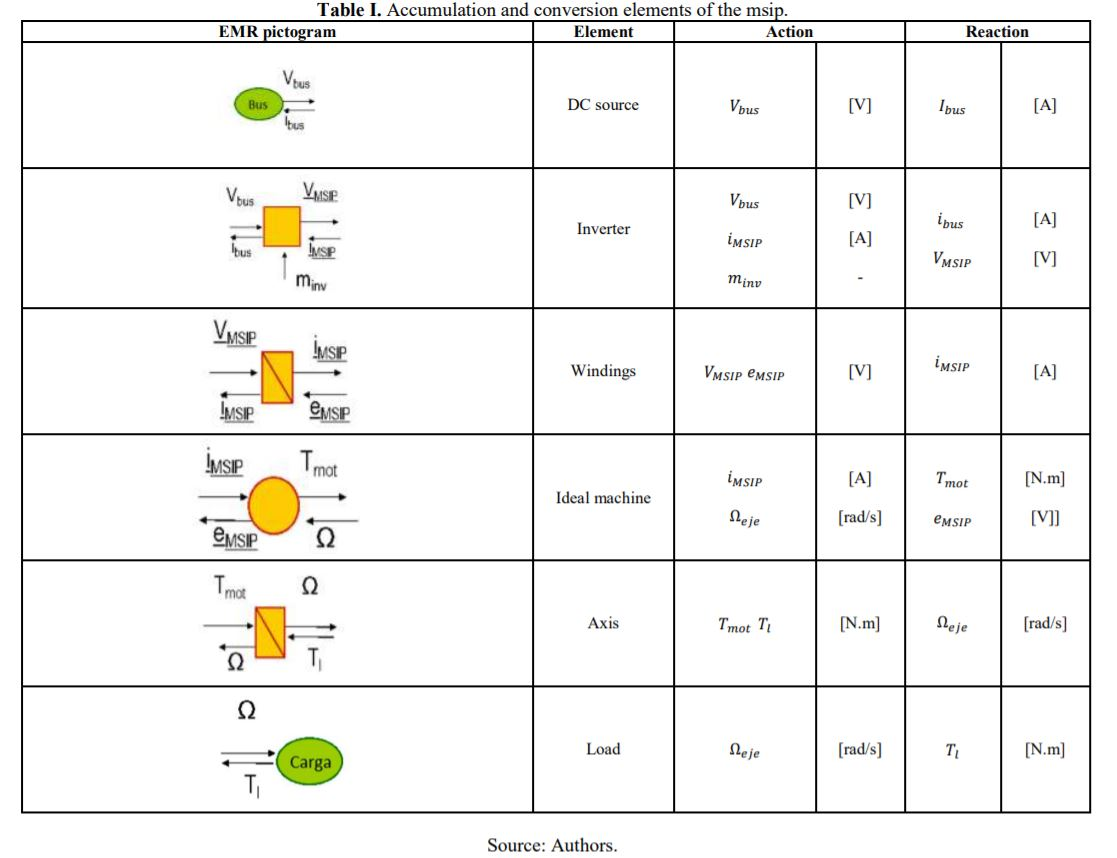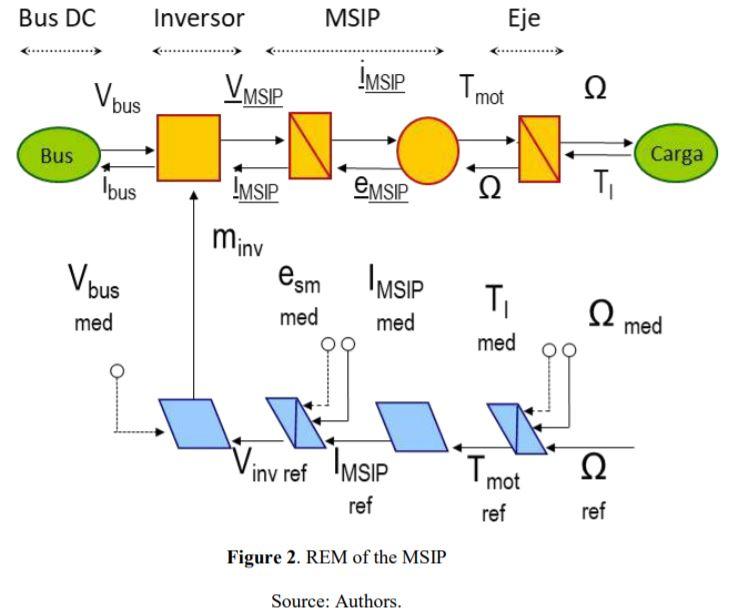It should be clarified that the accumulation block requires the speed of the machine, not for the exchange of energy, but to carry out the Park transformation. The speed of the machine is sent to the accumulation block, without appearing explicitly under REM. On the other hand, in the Simulink implementation, it will be explicitly displayed in another color (gray) to avoid confusion.

MSIP control structure

The control is based on the inversion rules of the local functions of the EMR elements, where it is required to define a reference input in each control block to establish the desired output. In this case, as shown in Figure 3, a reference signal of the speed of the machine to be followed is entered.

The accumulation elements induce state variables when they use integral causality, due to this, they cannot be physically inverted, and therefore, controllers are required to carry out indirect investments. Conversion elements by having time-independent relationships can be reversed directly .

Results and Discussion

The model implemented in Matlab Simulink (Figure 3) is validated by comparing two case studies with results obtained for two permanent magnet synchronous machines proposed in the literature  - . After validating the models, the control is evaluated by imposing variable operating conditions on the load torque, and it is sought to maintain a reference speed profile.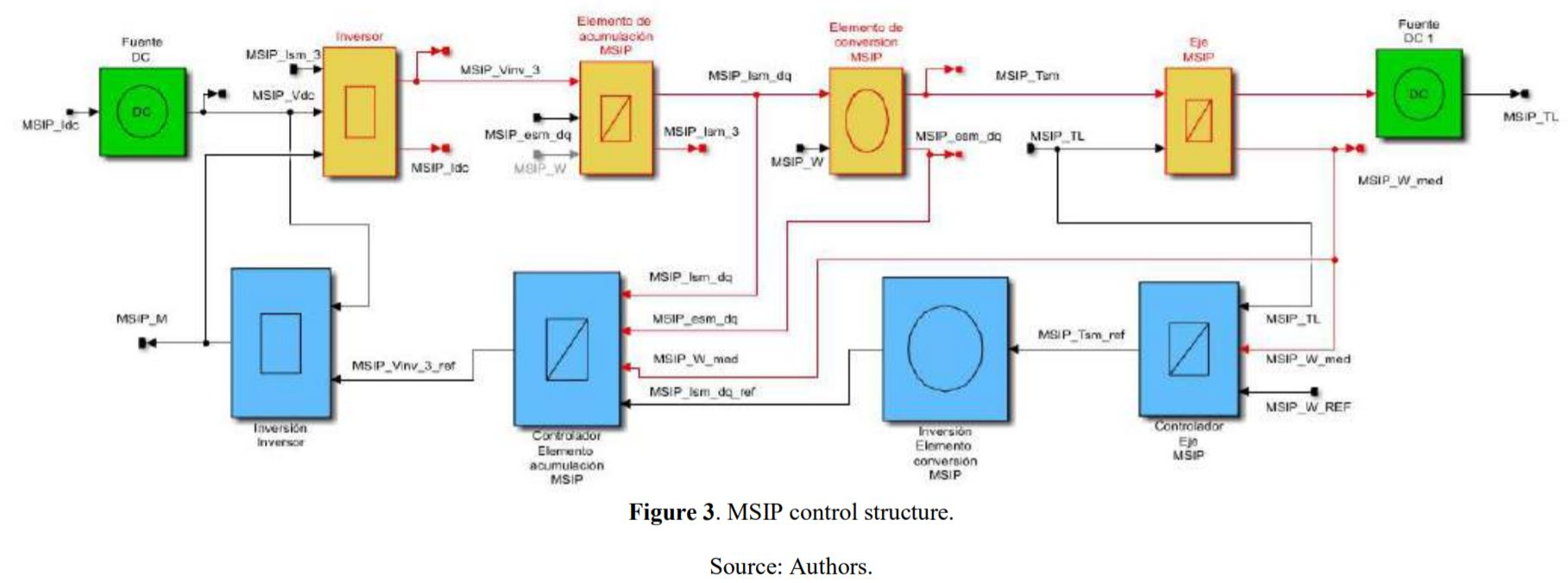Case study 1.

The first case study uses machine 1 presented in , whose parameters are presented in Table I.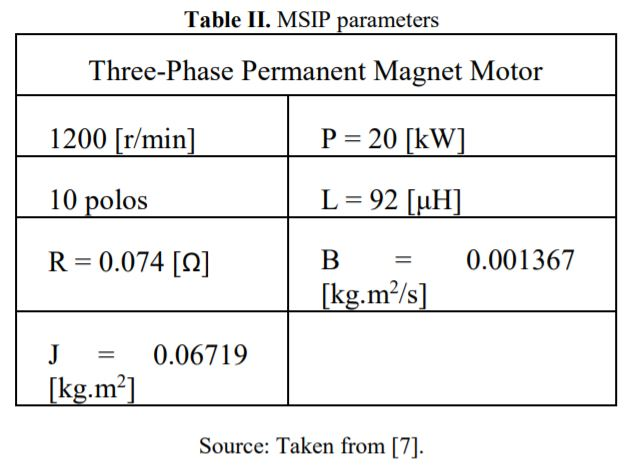Reference profiles for load torque (red) and speed (blue) are shown in Figure 4. A no-load start is simulated and then a load with a resistant torque of 8.45 [Nm] is added at 0.5 [s]. This shows that, from this EMR formalism, it is possible to control the speed of the machine based on the principles of action and reaction, causality, and the investment rules mentioned above.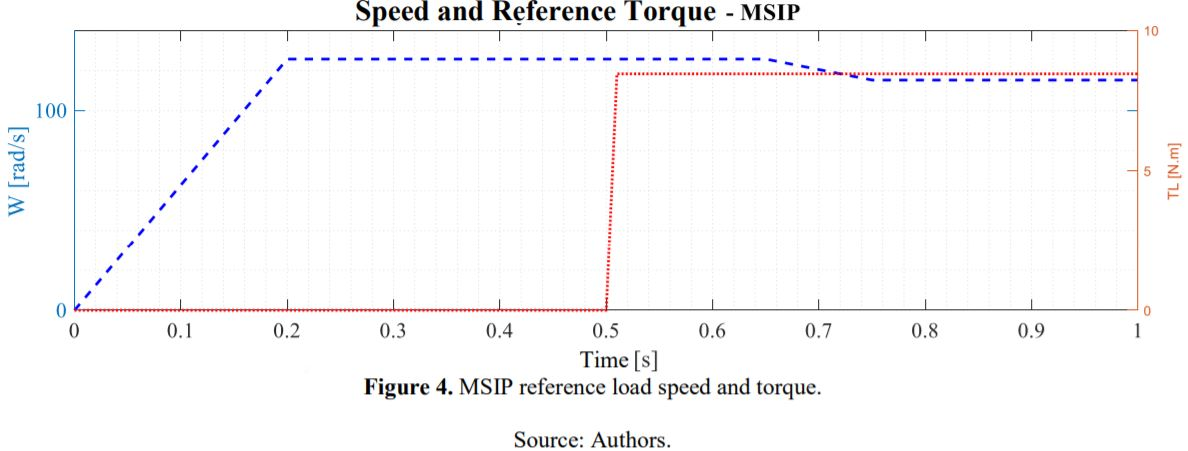Figure 5 shows the result obtained for the speed of the machine, where it can be seen that the correct monitoring of the required speed profile is carried out, even when the load is connected after 0.5 [s].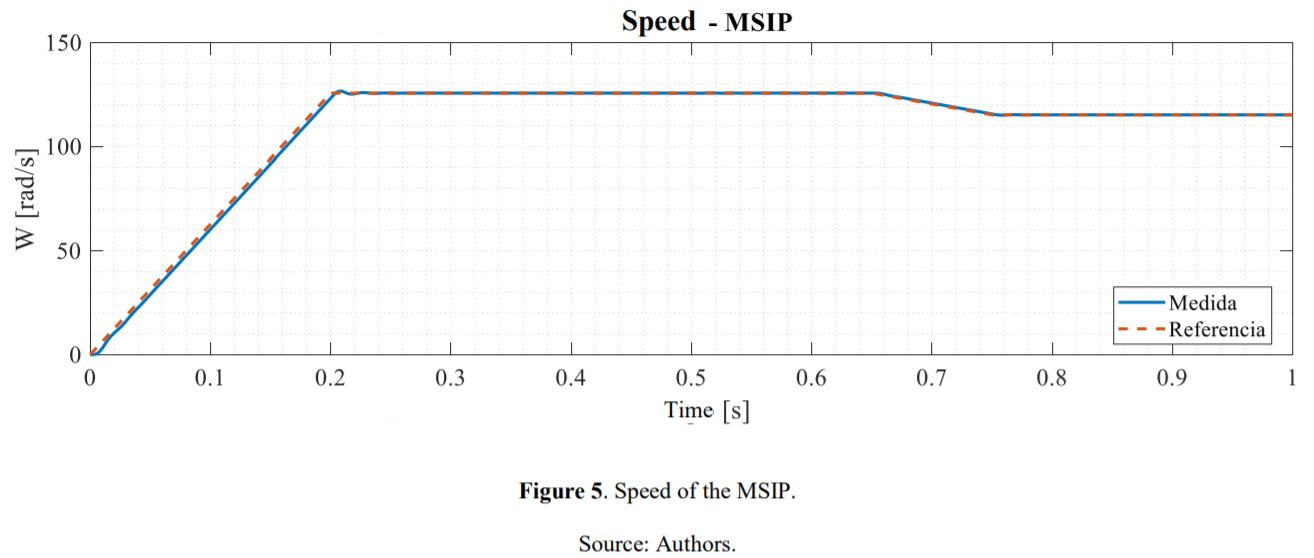Figure 6 presents the current and voltage of the MSIP. In this case, it is possible to observe the variations in the stator current and the voltage at the MSIP terminals when the variation in the load torque occurs at 0.5 [s] and in the speed at 0.65 [s], thus showing a normal behavior on the machine.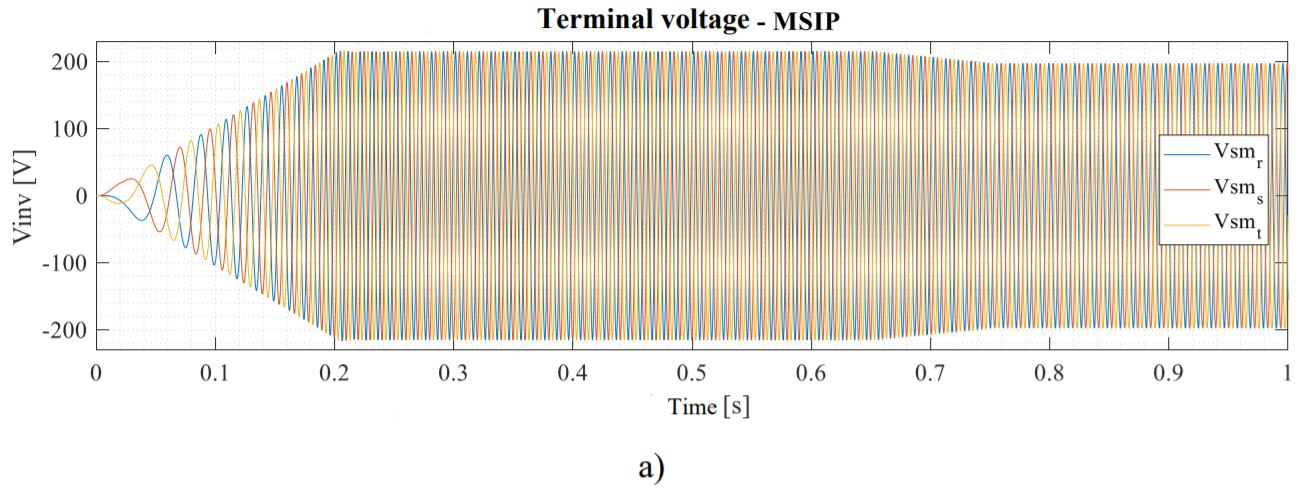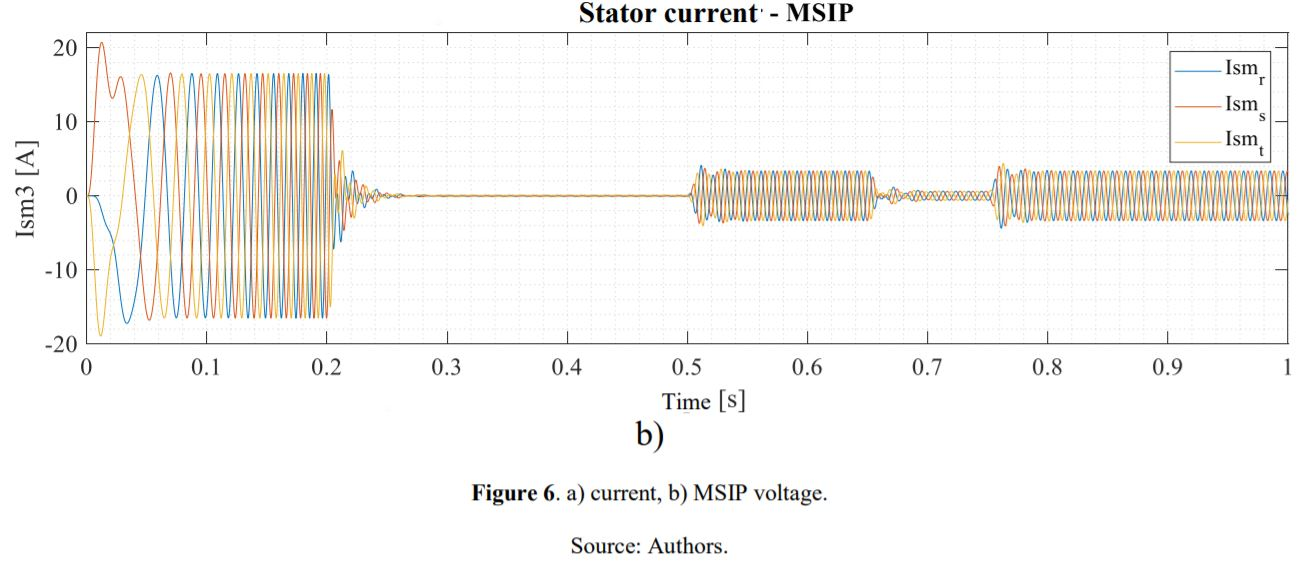In Figure 7 the previous results are shown with a zoom in the course of time in which the aforementioned variations are made to have a better visualization of the behavior of the main variables of the machine.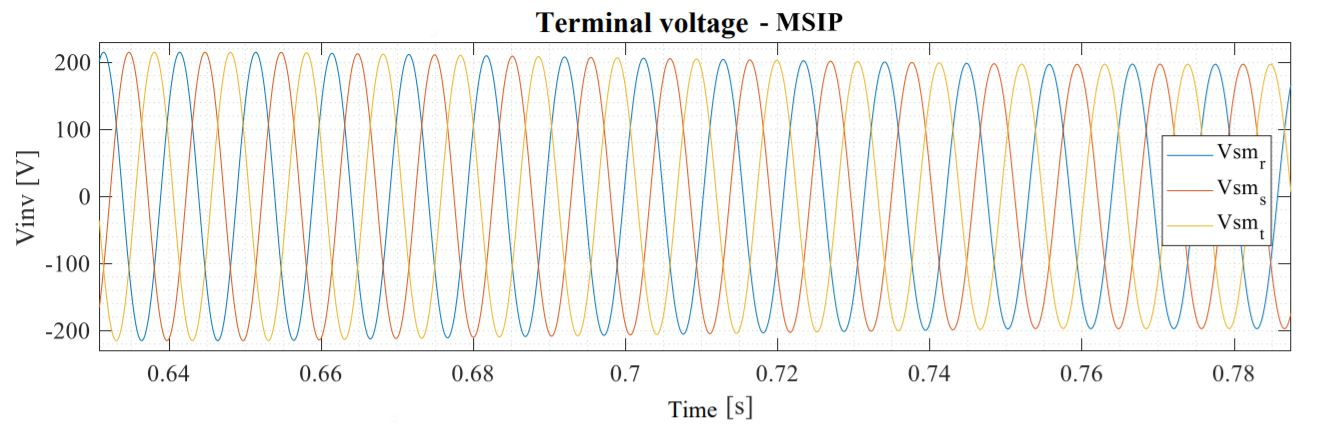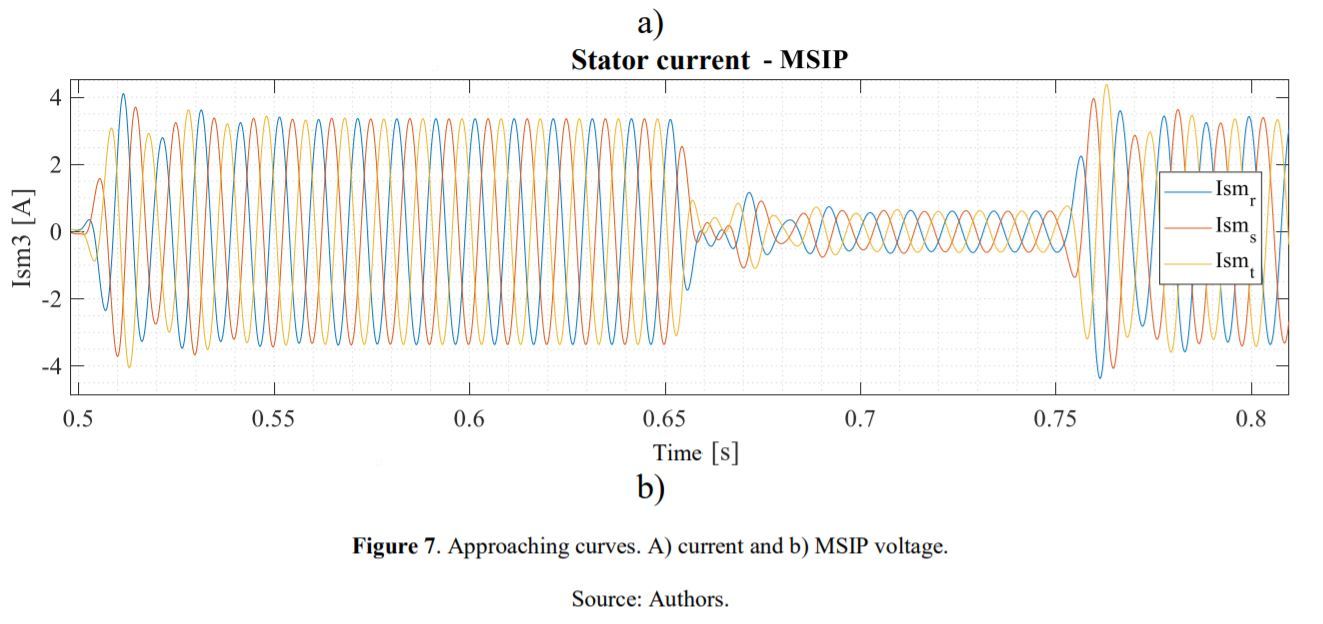Case study 2.

Below is another example of the use of the EMR formalism to control the speed of the machine proposed in . Its parameters are shown in Table III.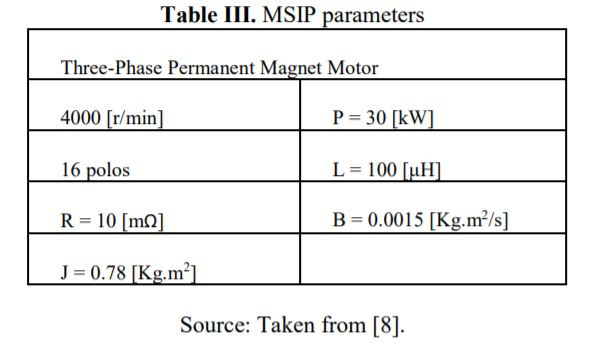The results obtained that are shown below in Figures 8-11 confirm that the macroscopic energy representation is an alternative for studying the models and control of different electromechanical machines and systems through the implementation of their pictograms, the investment rules, and the principle of causality.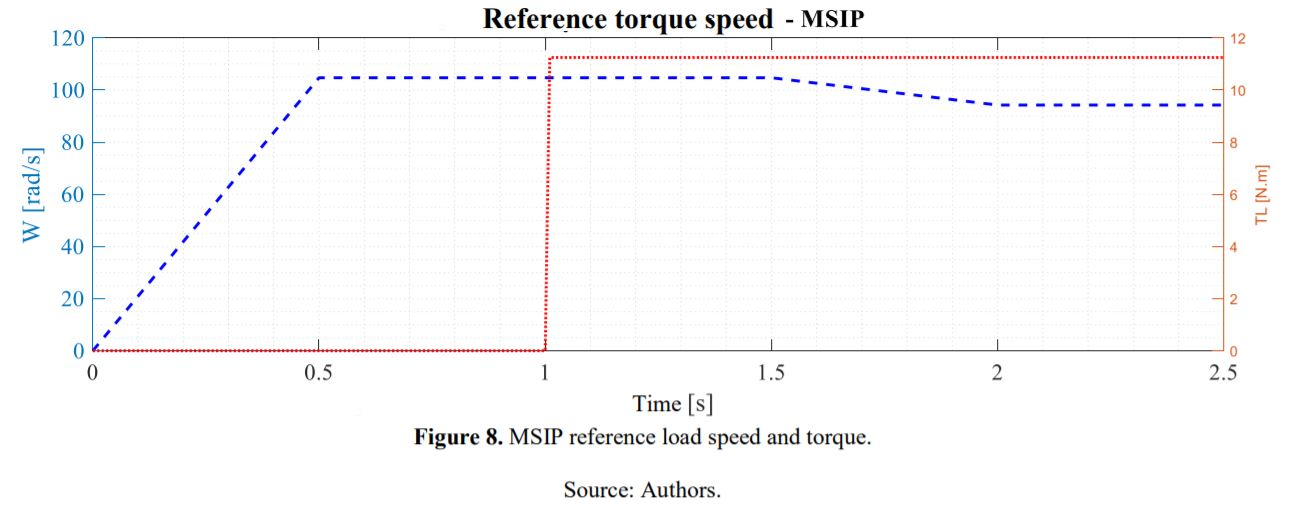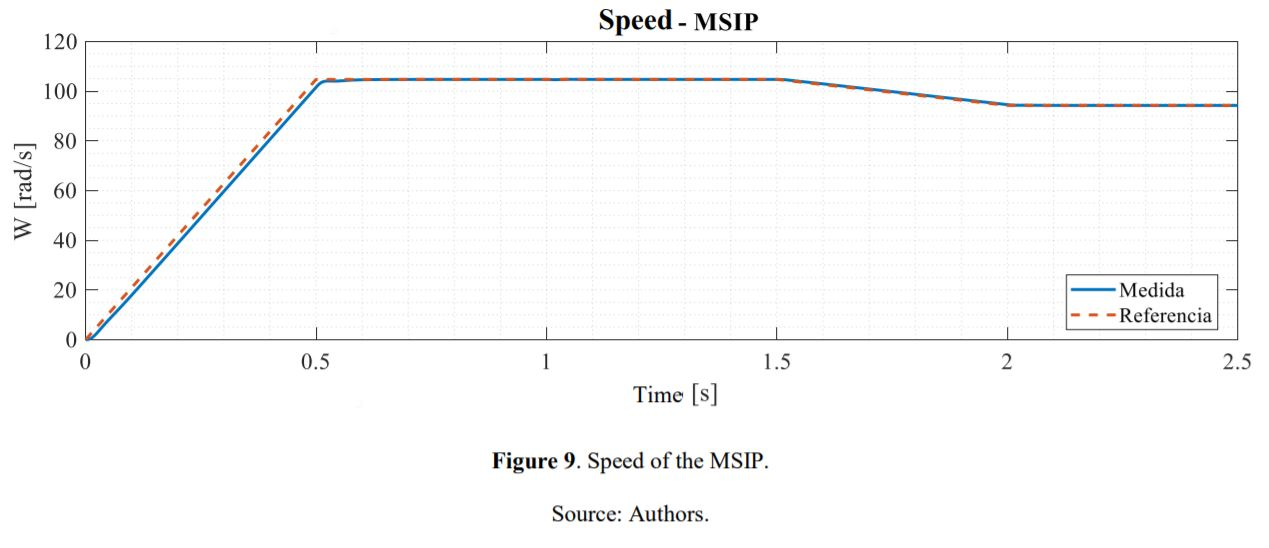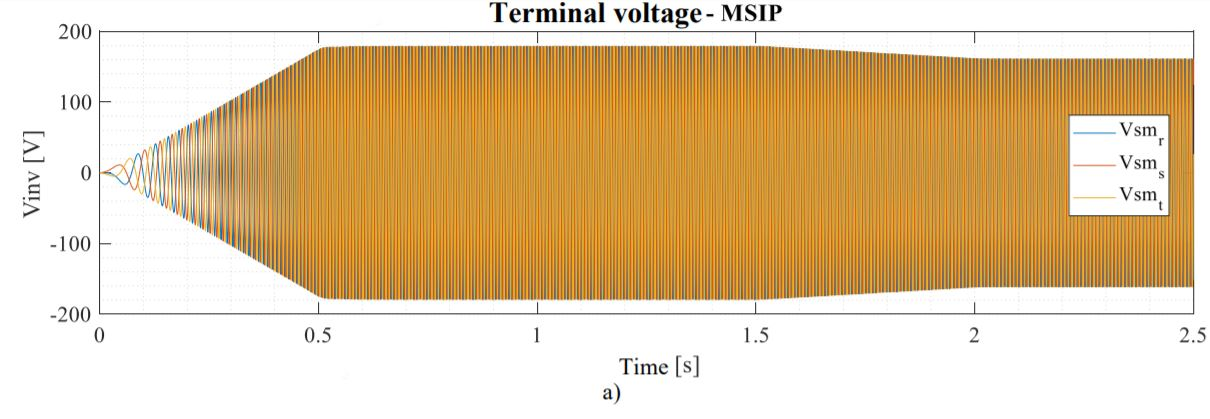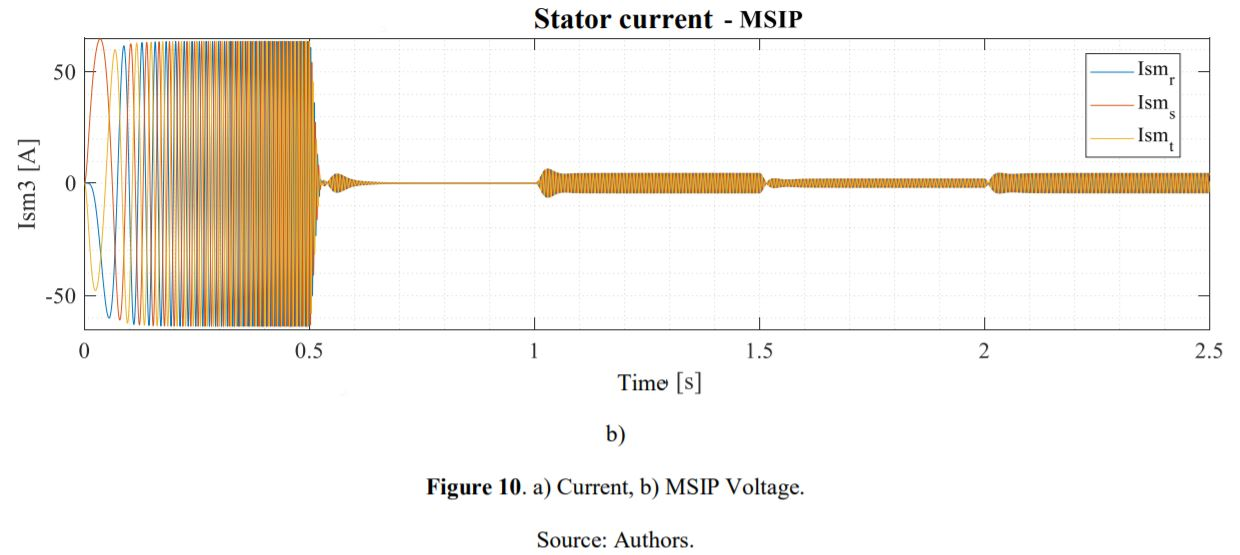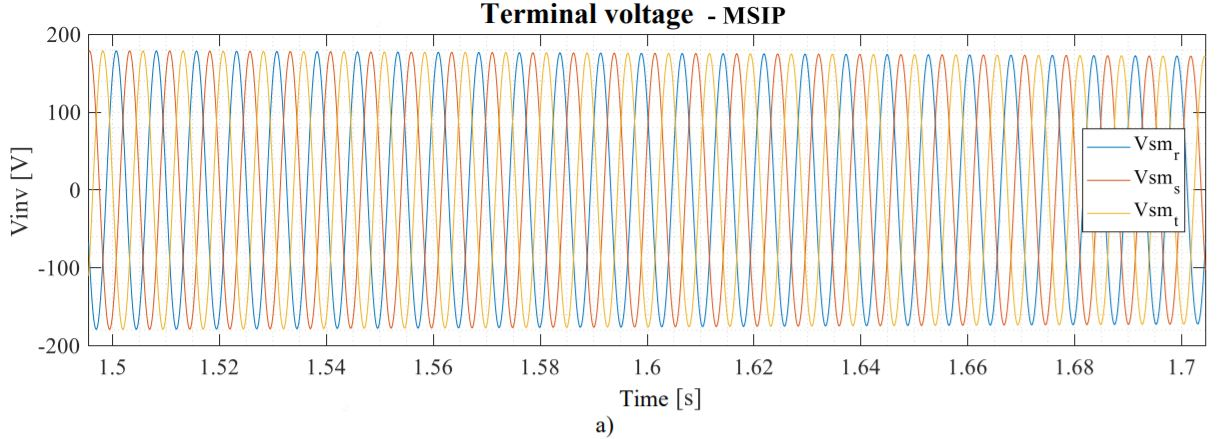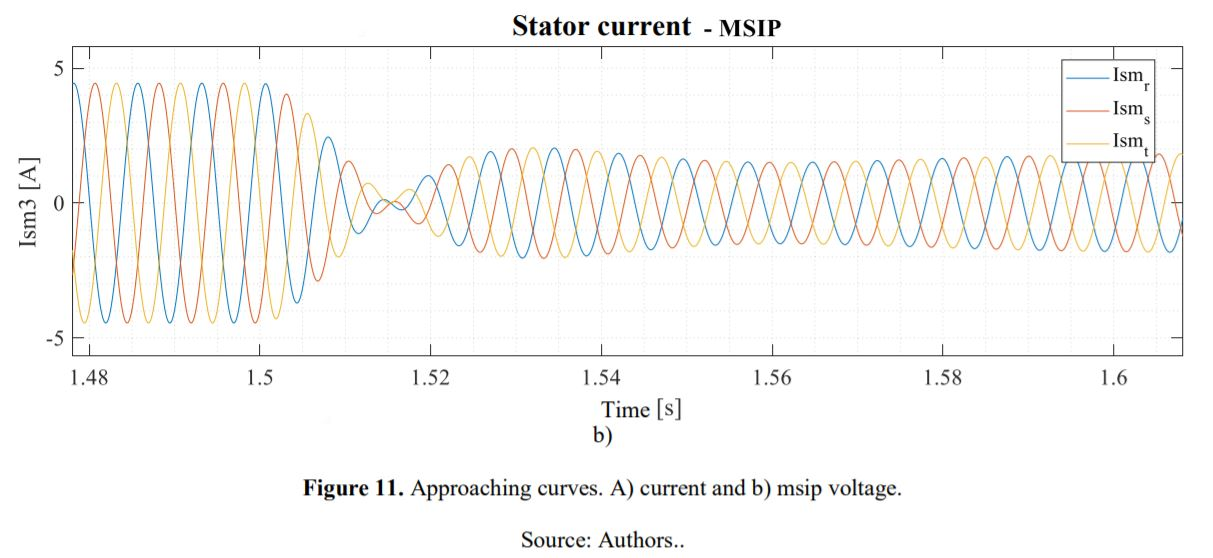Conclusions

The macroscopic energy representation provides a great facility to model and controls the MSIP and different electromechanical systems based on investment rules and integral causality.

The formalism represents an alternative for the simplified description of large processes that require a greater number of machines connected, and that due to their different pictograms can be analyzed in a more didactic way.

A simulation model is implemented in Matlab Simulink, which will be distributed at the request of interested researchers.

References

 A. Bouscayrol, P. Delarue and X. Guillaud, “Power strategies for maximum control structure of a wind energy conversion system with a synchronous machine”, Renew. Energy, vol. 30, no. 15, pp. 2273- 2288, 2005.

 A. Bouscayrol, P. Delarue, X. Guillaud and W. Lhomme, “Simulation of a Wind Energy Conversion System using Energetic Macroscopic Representation”, 2012 15th Int. Power Electron. Motion Control Conf., pp. DS3e.8-1-DS3e.8-6, 2012.

 A. Bouscayrol et al., “Teaching drive control using Energetic Macroscopic Representation”, initiation level Keywords.

 A. Bouscayrol et al., “Teaching drive control using Energetic Macroscopic Representation-expert level”, Power Electron. Appl. 2009. EPE ’09. 13th Eur. Conf., pp. 1-9, 2009.

 C. Mayet, M. Mejri, A. Bouscayrol, J. Pouget and Y. Riffonneau, “Energetic Macroscopic Representation and inversion-based control of the traction system of a hybrid locomotive”, 2012 IEEE Veh. Power Propuls. Conf. VPPC 2012, no. 1, pp. 491-496, 2012.

 Y. D. Wankam, P. Sicard and A. Bouscayrol, “Maximum control structure of a five-drive paper system using energetic macroscopic representation”, IECON Proc. Industrial Electron. Conf., no. Section V, pp. 5332-5337, 2006.

 J. Solano, “Energy management of a hybrid electric vehicle : an approach based on type-2 fuzzy logic To cite this version : HAL Id : tel-00699896 véhicule hybride lourd : approche par logique floue de type-2 Energy management of a hybrid electric vehicle : an approac”, 2012.

 F. D. E. Ingeniería and C. H. De Angelo, “CONTROL PARA MÁQUINAS DE CA DE IMANES PERMANENTES CON FEM ARBITRARIA, SIN SENSORES MECÁNICOS Cristian Hernán De Angelo”, 2004.

 J. Hautier, “The Causal Ordering Graph A tool for system modelling and control law synthesis”, Laboratoire d’Electrotechnique et d’Electronique de Puissance de Lille, ENSAM, 2014.

 P. Barre et al., “Inversion-based control of electromechanical systems using causal graphical descriptions To cite this version : HAL Id : hal-01109564 Science Arts & Métiers ( SAM )”, 2015.